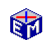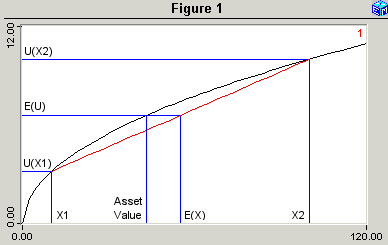Utility-Based Valuation of Risk

The most direct way to place an economic value on a risky asset is to observe the value of that asset in the financial markets, attributing the difference between the observed price and the price that might prevail without risk to the value of the risk.

Economics also studies the theory of risk valuation for agents with curved utility functions.  The theory shows that, if an agent's utility function features diminishing marginal utility of money, then that agent will be risk averse.  We can quantify the degree of that risk aversion for particular risky assets.Model Link:  Utility-Based Valuation of Risk
Printable PDF ExercisesThis EconModel application studies a simple numerical example that captures the flavor of the theory.  An agent contemplates an asset with two possible values, X1 and X2.  The probabilities of these values are 1-P and P.

To develop an intuitive understanding of this theory, the EconModel application lets the user change X1, X2, and P and redraw the diagram.  These steps will show how the value of the risk depends on the spread between the good and bad outcomes and the probabilities of those outcomes.

Classic Economic Models

Macroeconomics

Introduction
Overview of Macro Models

Microeconomics

Introduction
Overview of Micro Models

Resources